Need help with econocharts?
Click the “chat” button below for chat support from the developer who created it, or find similar developers for support.R-CoderDotCom193 Stars39 ForksOther61 Commits7 Opened issues#### Description

Microeconomics/macroeconomics charts in ggplot2!
?

#### Need anything else?#### Contributors list# 213,441
R
HTML
Shell
ggplot2...
54 commits

# econocharts package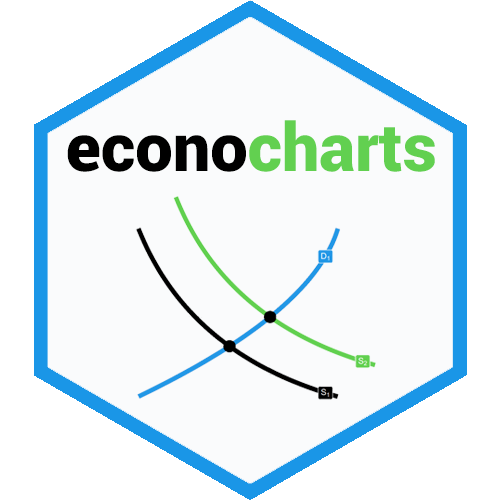This package allows creating microeconomics or macroeconomics charts in R with simple functions. This package inspiration is reconPlots by Andrew Heiss.

THE PACKAGE IS UNDER HEAVY DEVELOPMENT. WORK IN PROGRESS. You can suggest ideas by submitting an Issue or contributing submitting Pull Requests.

## TODO

• [ ] Finish documentation
• [x] Price control (in
`sdcurve`
function)
• [ ] Allow drawing custom functions
• [ ] Add graph for budget constraints
• [ ] Fix
`linecol`
argument
• [x] Tax graph
• [ ] Shade producer and consumer surplus
• [ ] Add Edgeworth box
• [ ] General equilibrium (suggested by Ilya)
• [x] Prospect theory value function (suggested by @brshallo)
• [x] Neoclassical labor supply (suggested by @hilton1)

## Installation

### GitHub

```# Install the development version from GitHub:
# install.packages("devtools")
devtools::install_github("R-CoderDotCom/econocharts")
```

### CRAN

The package will be on CRAN as soon as possible

## Supply

```supply() # Default plot
```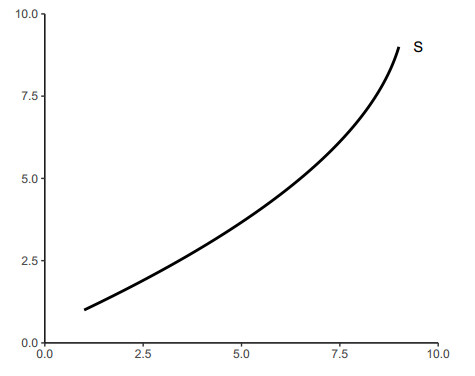```supply(ncurves = 1,          # Number of supply curves to be plotted
type = "line",         # Type of the curve
x = c(2, 4, 5),        # Y-axis values where to create intersections
linecol = 2,           # Color of the curves
geom = "label",        # Label type of the intersection points
geomfill = "pink",     # If geom = "label", is the background color of the label
main = "Supply curve") # Title of the plot
```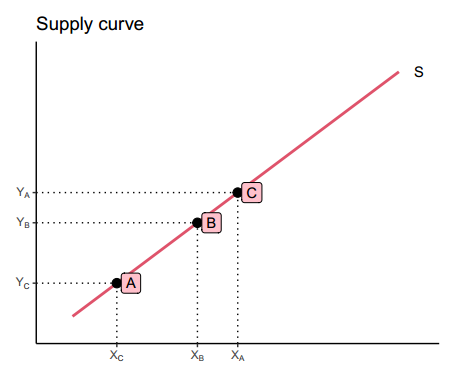```supply(ncurves = 3, # Three supply curves
xlab = "X",  # X-axis label
ylab = "Y",  # Y-axis label
bg.col = "lightblue") # Background color
```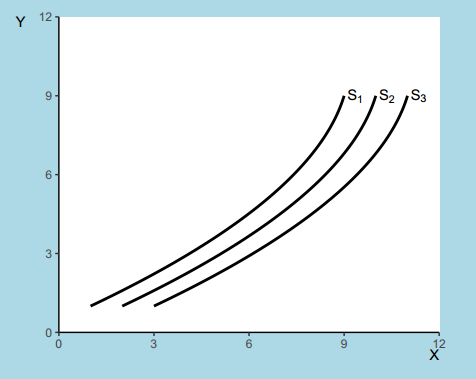## Demand

```demand(x = 3:6,  # Intersections
generic = FALSE) # Axis values with the actual numbers
```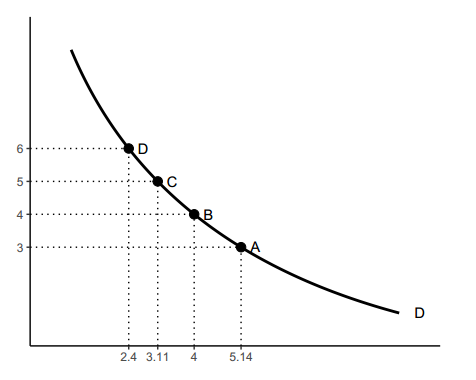```demand(main = "Demand", # Title
sub = "curve",   # Subtitle
xlab = "X",      # X-axis label
ylab = "Y",      # Y-axis label
names = "D",  # Custom name for the curve
geomcol = 2)     # Color of the custom name of the curve
```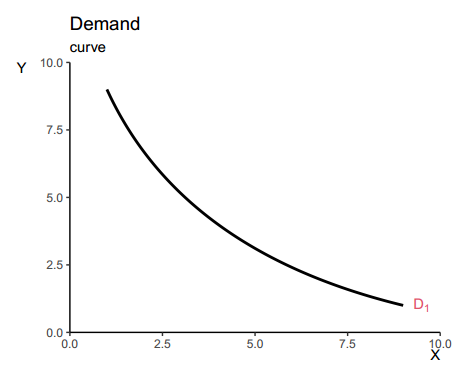## Supply and demand

```sdcurve() # Default supply and demand plot
```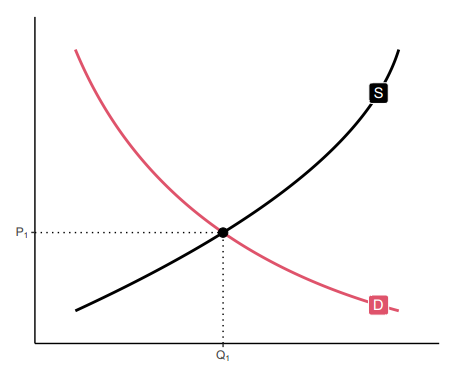```# Custom data
supply1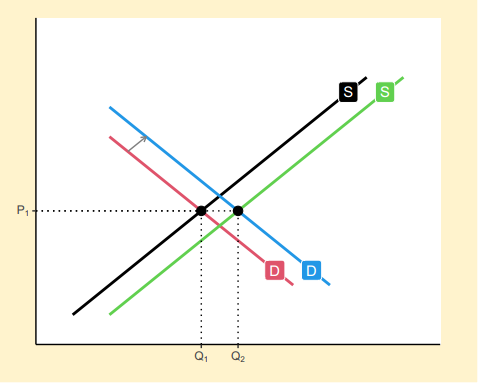Neoclassical labor supply
neolabsup(x = c(2, 3, 5, 7), xlab = "Quantity of\n labor supplied", ylab = "Wage rate")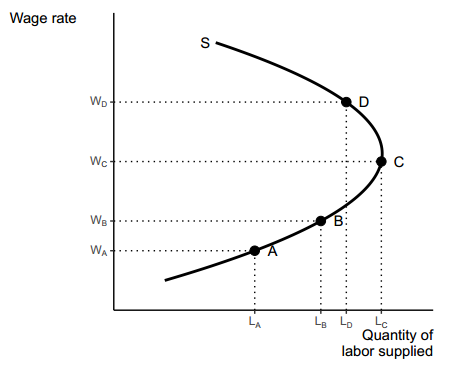Indifference curves
indifference() # Default indifference curve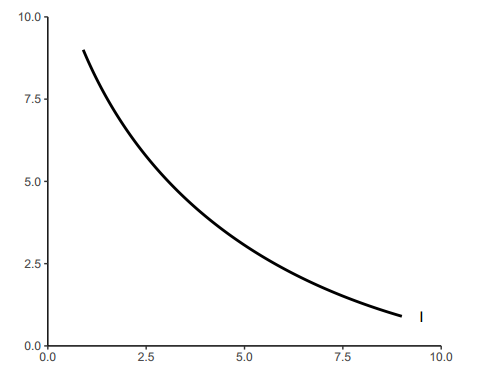indifference(ncurves = 2,  # Two curves
x = c(2, 4),  # Intersections
main = "Indifference curves",
xlab = "Good X",
ylab = "Good Y",
linecol = 2,  # Color of the curves
pointcol = 2) # Color of the intersection points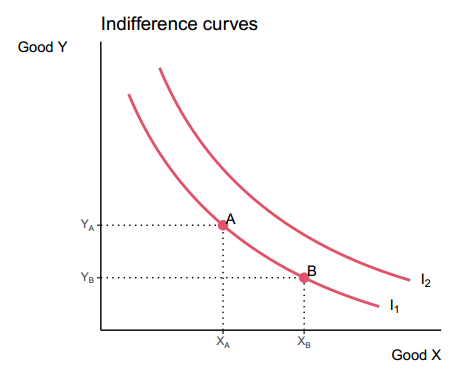p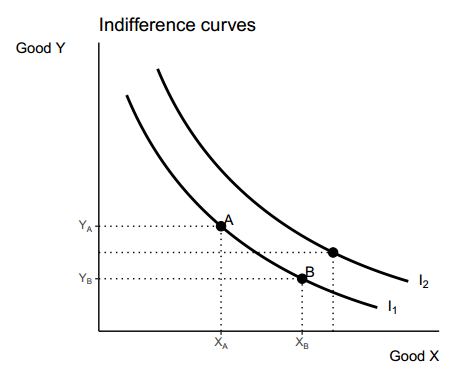indifference(ncurves = 2,    # Two curves
type = "pcom",  # Perfect complements
main = "Indifference curves",
sub = "Perfect complements",
xlab = "Good X",
ylab = "Good Y",
bg.col = "#fff3cd", # Background color
linecol = 1)  # Color of the curve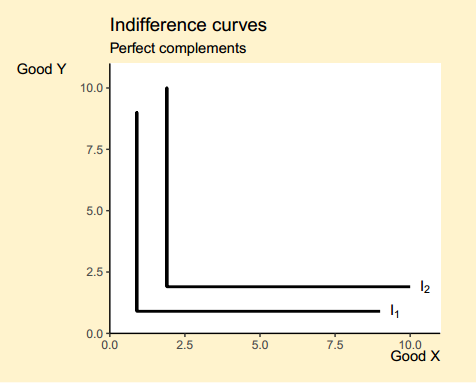indifference(ncurves = 5,     # Five curves
type = "psubs",  # Perfect substitutes
main = "Indifference curves",
sub = "Perfect substitutes",
xlab = "Good X",
ylab = "Good Y",
bg.col = "#fff3cd", # Background color
linecol = 1) # Color of the curve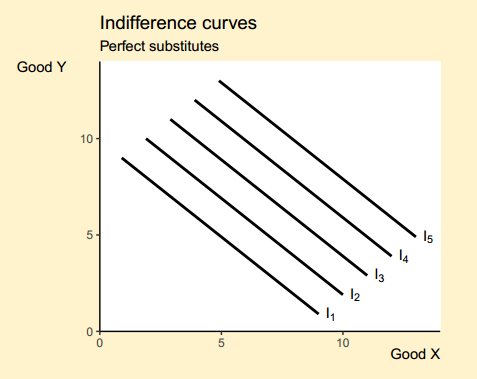Production–possibility frontier
ppf(x = 1:6, # Intersections
main = "PPF",
geom = "text",
generic = TRUE, # Generic axis labels
xlab = "X",
ylab = "Y",
labels = 1:6,
acol = 3)\$p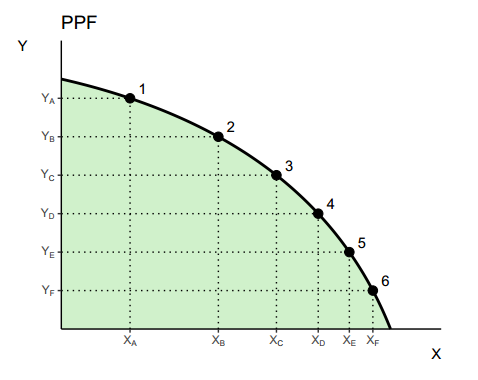p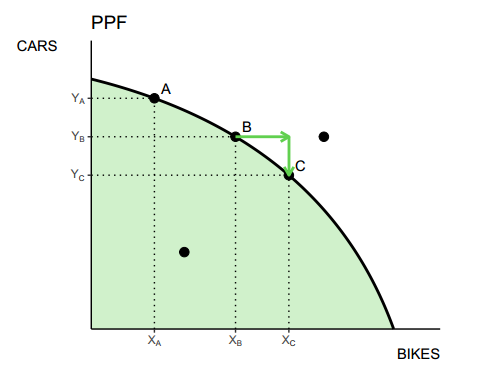Tax graph

Original function by Andrew Heiss.
# Data
demand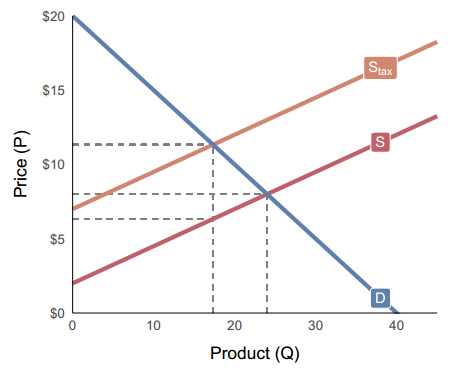tax_graph(demand, supply, supply_tax, shaded = TRUE)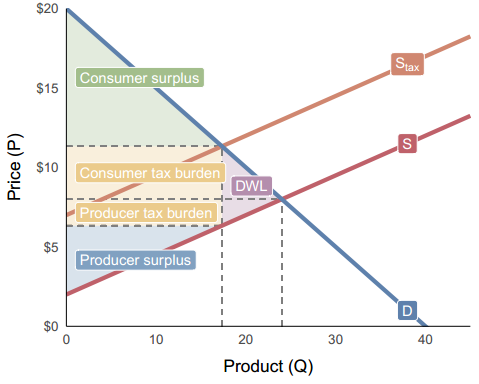Prospect theory value function
ptvalue(sigma = 0.3,
lambda = -2.25,
col = 2,                  # Color of the curve
xint = seq(0, 75, 25),    # Intersections
xintcol = 4,              # Color of the intersection segments
ticks = TRUE,             # Display ticks on the axes
xlabels = TRUE,           # Display the X-axis tick labels
ylabels = TRUE,           # Display the Y-axis tick labels
by_x = 25, by_y = 50,     # Axis steps
main = "Prospect Theory Value Function")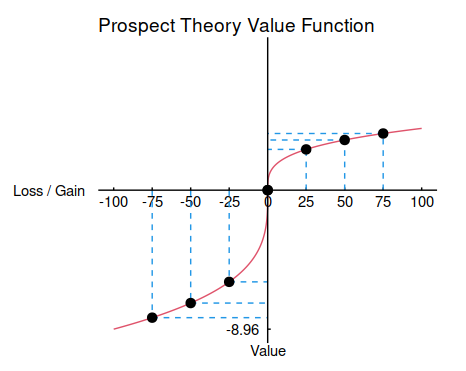Laffer curve
laffer(ylab = "T", xlab = "t",
acol = "lightblue", # Color of the area
pointcol = 4)       # Color of the maximum point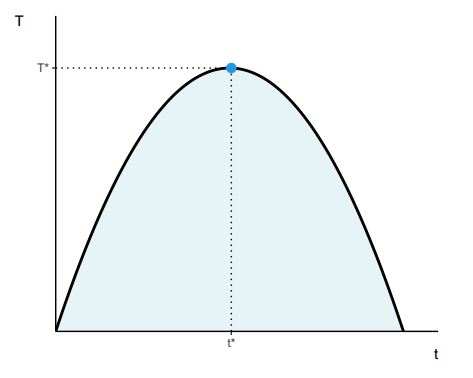laffer(xmax = 20, # Modify the curve
t = c(3, 6, 9), # Intersections
generic = FALSE,
ylab = "T",
xlab = "t",
acol = "lightblue", # Color of the area
alpha = 0.6,        # Transparency of the area
pointcol = 4)       # Color of the maximum point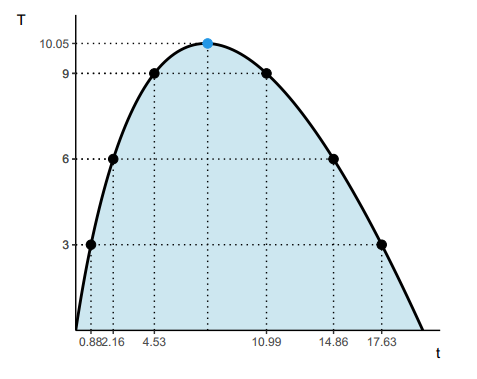Intersections

The functions above can have a limited functionality if you want a fully customized plot. The curve_intersection function allows you to calculate the intersection points between two curves. You can use this function to create your custom charts.

Credits to Andrew Heiss for this function and examples.

Curved Bézier lines with empirical data
# Curves
curve1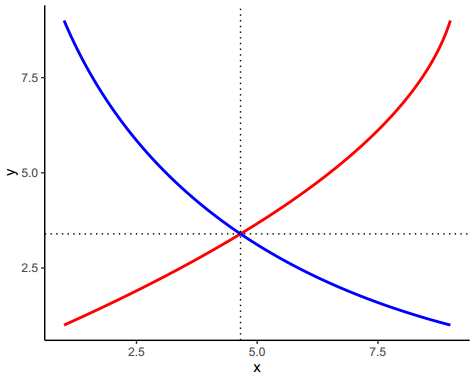Curved lines defined with functions

Specify a X-axis range and set empirical = FALSE.
# Define curves with functions
curve1Citation
To cite package ‘econocharts’ in publications use:

José Carlos Soage González and Andrew Heiss (2020). econocharts: Microeconomics and Macroeconomics Charts Made with 'ggplot2'. R package version 1.0.
https://r-coder.com/, https://r-coder.com/economics-charts-r/.
A BibTeX entry for LaTeX users is
@Manual{,
title = {econocharts: Microeconomics and Macroeconomics Charts Made with 'ggplot2'},
author = {José Carlos {Soage González} and Andrew Heiss},
year = {2020},
note = {R package version 1.0},
url = {https://r-coder.com/, https://r-coder.com/economics-charts-r/},
}

Social Media

```